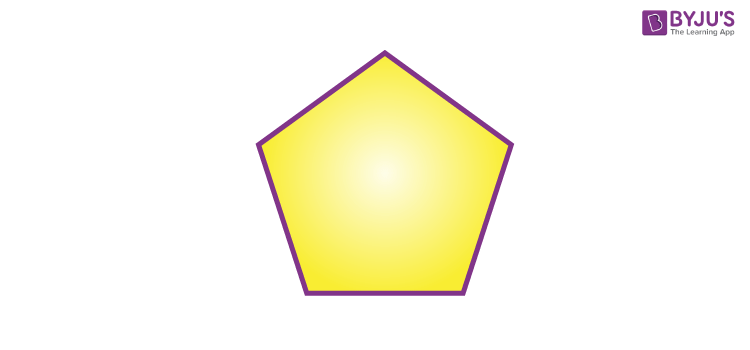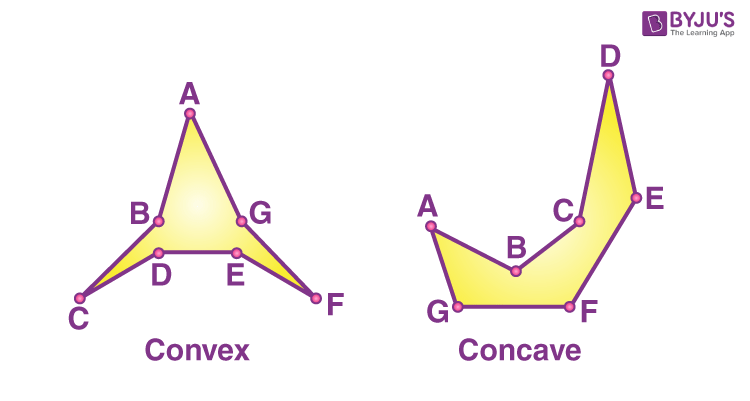Checkout JEE MAINS 2022 Question Paper Analysis : Checkout JEE MAINS 2022 Question Paper Analysis :

# Convex Polygon

A polygon is called a convex polygon when no line segments between the points, goes inside. The boundaries of the convex polygon do not go inside and all the vertices are pointed outside away from the center.

The interior angles of a convex polygon are less than 180°. Regularly, a polygon is firmly convex, if each line segment with two nonadjacent vertices of the polygon is strictly internal to the polygon but on its endpoints. Each non-fragment triangle is definitely convex.In the above diagram, the polygon shown is a convex polygon.

## Definition

The measures of the interior angles in a convex polygon are strictly less than 180 degrees. A regular polygon is always convex in nature. Convex polygons are the exact inverse of concave polygons. The vertices of a convex polygon always point outwards. Here, the difference between the convex polygon and concave polygon is given below:## What is Regular Convex Polygon?

A regular convex polygon is a polygon where each side is of the same length, and all the interior angles are equal and less than 180 degrees. The vertices and sides are evenly spread around a central point.

Example: A square is a regular convex polygon.

## Properties

The properties of the convex polygon are as follows:

• The interior angle of a convex polygon is strictly less than 180°.
• A polygon, with at least one interior angle, is greater than 180° is called a non-convex polygon or concave polygon
• Sum of all the interior angles of a polygon of n sides = (n – 2)180°.
• The diagonals of the convex polygon lie completely inside the polygon.
• Area of convex polygon can be determined by dividing the polygon into triangles and then finding the area of each triangle and summing up them.

## Convex Polygon Formula

The formula to find the area of a regular convex polygon is given as follows:

If the convex polygon has vertices (x1, y1), (x2, y2), (x3, y3),…….., (xn, yn), then the formula for the area of the convex polygon is

$$\begin{array}{l}A = \frac{1}{2}|\begin{pmatrix} x_{1} & x_{2}\\ y_{1} & y_{2} \end{pmatrix} + \begin{pmatrix} x_{2} & x_{3}\\ y_{2} & y_{3} \end{pmatrix} + ……..+ \begin{pmatrix} x_{n} & x_{1}\\ y_{n} & y_{1} \end{pmatrix}|\end{array}$$

## Convex Polygon Interior Angles

The interior angles of a polygon are defined as the angles inside the polygon. A polygon has the same number of interior angles as it does the sides. For example, if a polygon is quadrilateral, then the number of interior angles of a polygon is four. If a polygon is a pentagon, then the number of interior angles is five, and so on. We know that the polygon sum formula states that, for any n-polygon, the interior angles sum up to  (n – 2)180°.

Suppose, the number of sides of a convex polygon is 10, then, the sum of interior angles will be:

(10 – 2) x 180°

8 x 180°

= 1440°

Once if the sum of the interior angles of a convex polygon is known, we can easily find one of its interior angles when all the sides are congruent. It can be found by dividing the sum of the interior angles by the total number of sides.

## Convex Polygon Exterior Angles

The exterior angle of a polygon is defined as the angle formed bt extending the sides of a polygon. The exterior angle sum theorem states that the sum of the exterior angles of a convex polygon is 360°. If a convex polygon is regular with “n” number of sides, then each exterior angle of a convex polygon is measured as  360°/n.

## Solved Example

Question 1: Find the area of the polygon whose vertices are (5, 7), (9, 2) and (-4, 8)

Solution:

Given: The vertices are: (5, 7), (9, 2) and (-4, 8)

Here , (x1, y1) = (5, 7)

(x2, y2) = (9, 2)

(x3, y3) = (-4, 8)

The formula to find the area of a convex polygon is

A = ½ | (x1y2 – x2y1) + (x2y3 – x3y2) + (x3y1 – x1y3) |

A = ½ | (10-63) + (72 +8 ) (-40-28)|

A = ½ | -41|

A = ½ |41|

A = 41/2

Therefore, the area of the convex polygon is 41/2.

Question 2: A convex polygon has 44 diagonals, find the number of sides of his this polygon.

Solution: No. of diagonals in a polygon of n sides =n(n-3)/2

n(n-3)/2 = 44

n(n−3)=88

n(n−3)=11(11−3)

∴n=11

∴ No. of sides =11

Stay tuned with BYJU’S – The Learning App and also explore more Maths-related videos.

## Frequently Asked Questions – FAQs

### How many sides does a convex polygon have?

A convex polygon is a closed shape, where none of the vertices are pointed inward. When a line crosses the convex polygon, it intersects at most the two sides of the polygon. Any polygon such as pentagon, hexagon, etc, whose vertices are pointed outside the center of the polygon, are convex.

### How can we determine if a polygon is convex or concave?

If the interior angles of of the polygon are less than 180 degrees, then the polygon is convex. But if any one of the interior angles is more than 180 degrees, then the polygon is concave.

### What is the shape of convex polygon?

A convex polygon can be regular or irregular. It is a closed polygon whose interior angles are less then 180 degrees. No vertices of convex polygon are pointed inside.

### What are the examples of convex polygon?

Kite, Pentagon, Hexagon, Heptagon, etc. are examples of convex polygon.

### What are the types of polygon based on shape?

Based on the shape of polygon, the types of a polygon are convex and concave, regular and irregular, simple and complex.